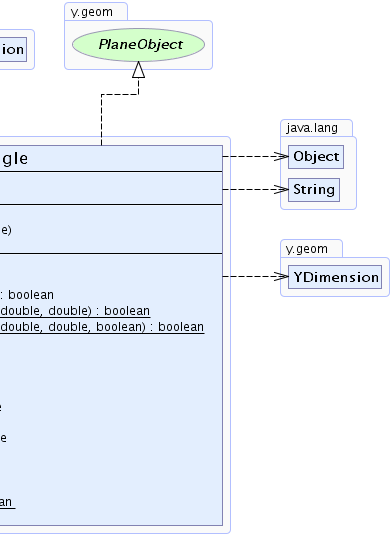Search this API

## y.geom Class YRectangle

```java.lang.Objecty.geom.YDimensiony.geom.YRectangle
```
All Implemented Interfaces:
java.lang.Comparable, PlaneObject

`public class YRectangleextends YDimensionimplements PlaneObject`

This class defines a rectangle and provides utility methods for it.Field Summary
` double` `x`
The x coordinate of the upper left corner.
` double` `y`
The y coordinate of the upper left corner.

Fields inherited from class y.geom.YDimension
`height, width`

Constructor Summary
`YRectangle()`
Creates a new rectangle with upper left corner (0,0) and size (0,0).
```YRectangle(double x, double y, double width, double height)```
Creates a new rectangle with given upper left corner and size.
```YRectangle(YPoint pos, YDimension size)```
Creates a new rectangle with given upper left corner and size.

Method Summary
` int` `compareTo(java.lang.Object o)`

` boolean` ```contains(double x, double y)```
Checks whether or not this `YRectangle` contains the given point.
` boolean` ```contains(double x, double y, double width, double height)```
Checks whether or not this `YRectangle` contains the rectangle defined by the given frame.
`static boolean` ```contains(double rx, double ry, double rw, double rh, double x, double y)```
Determines whether the specified rectangle contains the specified point.
`static boolean` ```contains(double rx, double ry, double rw, double rh, double x, double y, boolean closed)```
Determines whether the specified rectangle contains the specified point.
` boolean` `contains(YPoint p)`
Checks whether or not this `YRectangle` contains the given point.
` boolean` `contains(YRectangle p)`
Checks whether or not this `YRectangle` contains the given rectangle.
` boolean` `equals(java.lang.Object o)`
Tests a dimension to equality to another dimension.
` YRectangle` `getBoundingBox()`
Returns this object.
` double` `getEuclideanDistance(YRectangle other)`
Returns the Euclidean distance to the passed rectangle.
` YPoint` `getLocation()`
Returns coordinates of upper left corner.
` double` `getManhattanDistance(YRectangle other)`
Returns the Manhattan distance to the passed rectangle.
` double` `getX()`
Returns x-coordinate of upper left corner.
` double` `getY()`
Returns y-coordinate of upper left corner.
` int` `hashCode()`

`static boolean` ```intersects(YRectangle r1, YRectangle r2)```
Returns whether or not the given rectangles intersect.
` java.lang.String` `toString()`
Returns a string representation of this rectangle

Methods inherited from class y.geom.YDimension
`getHeight, getWidth`

Methods inherited from class java.lang.Object
`clone, finalize, getClass, notify, notifyAll, wait, wait, wait`

Field Detail

### x

`public final double x`
The x coordinate of the upper left corner.

### y

`public final double y`
The y coordinate of the upper left corner.

Constructor Detail

### YRectangle

`public YRectangle()`
Creates a new rectangle with upper left corner (0,0) and size (0,0).

### YRectangle

```public YRectangle(YPoint pos,
YDimension size)```
Creates a new rectangle with given upper left corner and size.

Parameters:
`pos` - upper left corner of the rectangle.
`size` - size of the rectangle.

### YRectangle

```public YRectangle(double x,
double y,
double width,
double height)```
Creates a new rectangle with given upper left corner and size.

Parameters:
`x` - x-coordinate of upper left corner of the rectangle.
`y` - y-coordinate of upper left corner of the rectangle.
`width` - width of the rectangle.
`height` - height of the rectangle.
Method Detail

### getManhattanDistance

`public double getManhattanDistance(YRectangle other)`
Returns the Manhattan distance to the passed rectangle. If they overlap the distance is 0.

Parameters:
`other` - the second rectangle.
Returns:
the distance to the given rectangle.

### getEuclideanDistance

`public double getEuclideanDistance(YRectangle other)`
Returns the Euclidean distance to the passed rectangle. If they overlap, the distance is 0.

Parameters:
`other` - the second rectangle.
Returns:
the distance to the given rectangle.

### getX

`public final double getX()`
Returns x-coordinate of upper left corner.

### getY

`public final double getY()`
Returns y-coordinate of upper left corner.

### getLocation

`public final YPoint getLocation()`
Returns coordinates of upper left corner.

### getBoundingBox

`public YRectangle getBoundingBox()`
Returns this object.

Specified by:
`getBoundingBox` in interface `PlaneObject`

### contains

```public boolean contains(double x,
double y)```
Checks whether or not this `YRectangle` contains the given point.

Parameters:
`x` - the x-coordinate of the point to check.
`y` - the x-coordinate of the point to check.
Returns:
`true` if the point lies inside the rectangle; `false` otherwise.

### contains

```public static boolean contains(double rx,
double ry,
double rw,
double rh,
double x,
double y)```
Determines whether the specified rectangle contains the specified point.

Parameters:
`rx` - the x-coordinate of the upper left corner of the rectangle.
`ry` - the y-coordinate of the upper left corner of the rectangle.
`rw` - the width of the rectangle.
`rh` - the height of the rectangle.
`x` - the x-coordinate of the point to check.
`y` - the x-coordinate of the point to check.
Returns:
`true` if the point lies inside the rectangle; `false` otherwise.

### contains

```public static boolean contains(double rx,
double ry,
double rw,
double rh,
double x,
double y,
boolean closed)```
Determines whether the specified rectangle contains the specified point.

Parameters:
`rx` - the x-coordinate of the upper left corner of the rectangle.
`ry` - the y-coordinate of the upper left corner of the rectangle.
`rw` - the width of the rectangle.
`rh` - the height of the rectangle.
`x` - the x-coordinate of the point to check.
`y` - the x-coordinate of the point to check.
`closed` - if `true`, all points on the border of the rectangle are considered to be contained.
Returns:
`true` if the point lies inside the rectangle; `false` otherwise.

### contains

`public boolean contains(YPoint p)`
Checks whether or not this `YRectangle` contains the given point.

### contains

`public boolean contains(YRectangle p)`
Checks whether or not this `YRectangle` contains the given rectangle.

### contains

```public boolean contains(double x,
double y,
double width,
double height)```
Checks whether or not this `YRectangle` contains the rectangle defined by the given frame.

### intersects

```public static boolean intersects(YRectangle r1,
YRectangle r2)```
Returns whether or not the given rectangles intersect.

### toString

`public java.lang.String toString()`
Returns a string representation of this rectangle

Overrides:
`toString` in class `YDimension`

### hashCode

`public int hashCode()`
Overrides:
`hashCode` in class `YDimension`

### equals

`public boolean equals(java.lang.Object o)`
Description copied from class: `YDimension`
Tests a dimension to equality to another dimension.

Overrides:
`equals` in class `YDimension`

### compareTo

`public int compareTo(java.lang.Object o)`
Specified by:
`compareTo` in interface `java.lang.Comparable`
Overrides:
`compareTo` in class `YDimension`